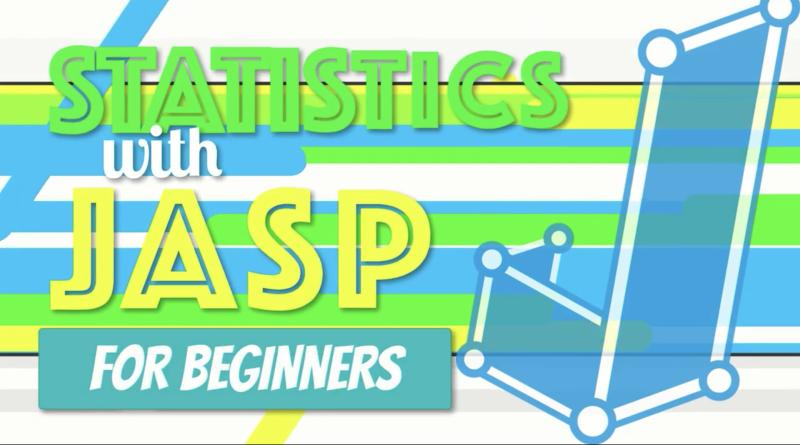# Prediction## Video: How to Do Simple Linear Regression in JAS

Video (23:18) from Research by Design in which, “Exploring our data about burnout and job satisfaction, we predict an outcome with a single variable using simple linear regression in JASP. I explain how regression works, then open an SPSS dataset in JASP. We explore the assumptions of homoscedasticity and linearity with a scatterplot and normality …## Tutorial: Regression (WISE)

Online tutorial that guides students through a research study and data analysis using regression. There are 4 modules total, with the first three being most appropriate for an introductory course. Module 3 is short and could be used as an in-class demonstration. Module 4 is more advanced. During the tutorial students will start with a …## Lecture Slides: Predicting Job Performance (Multiple Regression)

This is an example (created by Dr. Alisa McArthur) looking at predictors of job performance that shows simple multiple regression using SPSS which illustrates how multicollinearity can lead to results that are inconsistent and difficult to interpret. It includes both a PowerPoint as well as hard copy for students to help them get more comfortable …